1623698160

Selection Sort

In computer science, selection sort is an in-place comparison sorting algorithm. It has an O(_n_2) time complexity, which makes it inefficient on large lists, and generally performs worse than the similar insertion sort. Selection sort is noted for its simplicity and has performance advantages over more complicated algorithms in certain situations, particularly where auxiliary memory is limited.

Selection Sort:

var unOrderArray = [4,2,6,10,8]
Suppose we have array of unordered numbers(In this case even number, subset of natural numbers)
We have two option to sort this.
1\. Ascending order.
2\. Descending order.

#programming #sorting-algorithms #selection-sort #swift

Buddha Community1623975000

Selection Sort in Java

Introduction

Sorting data is a frequent problem in computer science. Given a collection of elements, the goal is to rearrange them in some order. Common examples are sorting an array alphabetically or from smallest to largest.

Sorted data is a lot easier to manipulate. Finding the largest or smallest element of an array can be done in constant time if the array is sorted. Searching for an element is a lot faster using algorithms such as Binary Search which rely on the assumption that the array is already sorted.

One of the simplest algorithms for sorting data is Selection Sort. It’s usually taught in beginner programming classes and tutorials to explain the concept of sorting, so we’ll keep this article very beginner-friendly.

#java #selection sort in java #selection sort1623698160

Selection Sort

In computer science, selection sort is an in-place comparison sorting algorithm. It has an O(_n_2) time complexity, which makes it inefficient on large lists, and generally performs worse than the similar insertion sort. Selection sort is noted for its simplicity and has performance advantages over more complicated algorithms in certain situations, particularly where auxiliary memory is limited.

Selection Sort:

var unOrderArray = [4,2,6,10,8]
Suppose we have array of unordered numbers(In this case even number, subset of natural numbers)
We have two option to sort this.
1\. Ascending order.
2\. Descending order.

#programming #sorting-algorithms #selection-sort #swift1593542940

Heap Sort Explained

As you may know, heapsort is also one of the most asked sorting algorithms besides mergesort and quicksort and today we’re going to talk about it and I’ll try my best to explain it in much simpler terms as for a beginner it can be quite complicated more so if one has less knowledge about heaps. But fear not I’ve got your back. So now let’s get into it.

Before moving on to heap sort you need to know about the following terminologies to properly understand the working of heapsort.

1. Heaps
2. Heap Property
3. Heapify

So let’s begin by explaining the terms stated above

1. Heaps

Heap data structure is a**_ complete binary tree that follows the heapify property_**. So what’s a complete binary tee you might ask?. A complete binary tree follows these conditions.

• All nodes in every level of the tree are filled except the leaf nodes.
• The nodes are as far left as possible.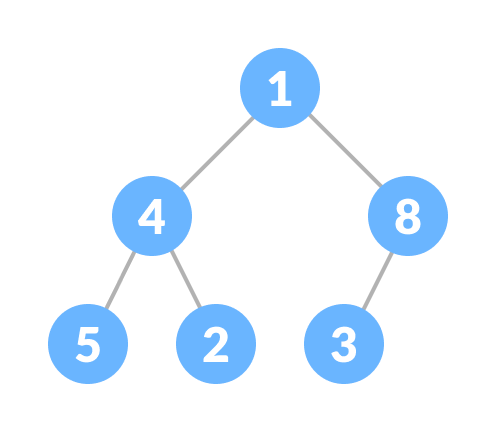Complete Binary Tree

The heap is shown below following the heap property and this is called Max heap. It has the same root and the child nodes but there is a slight difference. The Complete Binay Tree doesn’t care whether it’s root node is smaller or larger but it is of grave importance in case of the heap data structure.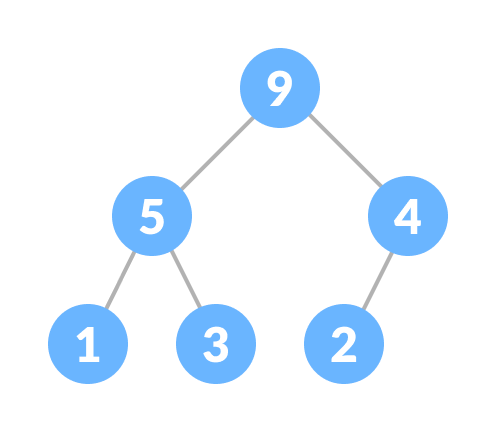Heap

2. Heap Property

While creating a heap it is essential to follow the heap property which states as follows:

• The heap where the parent node is always greater than the child nodes and provided that the root node is largest is called a Max-heap.Max Heap

• The heap in which the child node is always greater than it’s child nodes and the root node is the smallest node is called a Min-heap.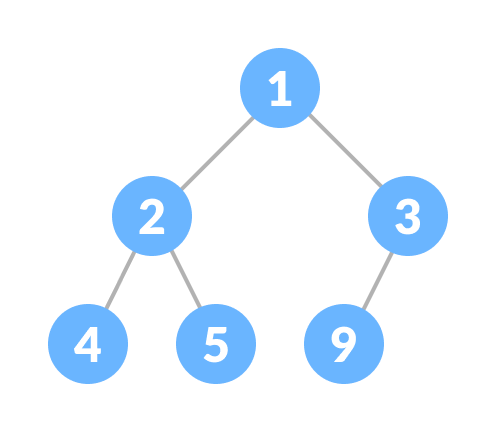Min heap

3. Heapify

Heapify is the** process of creating a heap data structure from a binary tree. **Now Heapify is the most important operation on a heap data structure as it helps to maintain the structure of the heap after several operations have been performed on the heap like(insertion, deletion). We will be using this operation a lot and it will come in handy while designing heap sort.

working of heapify is shown below

1. Select an input array.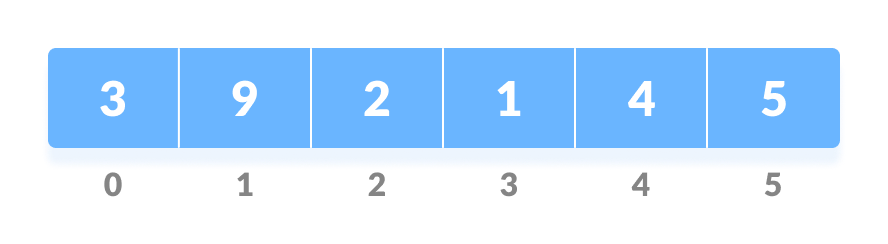Input array

2. Convert this array into a complete binary tree.

Complete binary tree created from the array

3. Create a heap from the binary tree.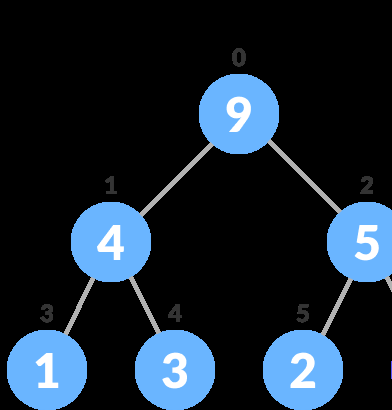After Heapify a max heap is created

Now that we’ve learned about all the important terms let’s start to create heap sort algorithm.

In a heap sort program, we’ll be given an unsorted array and we have to sort it. But heapsort works somewhat different than other sorting algorithms. By different, means the arrangement of each element after operations are performed. In this, the larger elements are sorted first in the last place then followed by the smaller elements, and at last, when there’s one element left that element would be the smallest and the last to go in it’s sorted place.

#sorting #sorting-algorithms #heap #heapsort #sort1592698920

Selection Sort Algorithm Visually Explained

The Selection Sort algorithm sorts an array by looking for the smallest item and moving it to the front of the list. That’s really all you have to know. Let’s jump into an example.
Selection Sort starts by setting the first value as the minimum value.
It then starts comparing it with each value in the array. If a lower value is found, the minimum value is updated, and the traversal continues until the end of the array is reached. During the first comparison, the value at index 1 is compared to the minimum value.

#programming #algorithms #computer-science #sorting-algorithms #selection-sort1600173300

Selection Sort In Java Example | Java Selection Sort Program

Sorting means to put all the elements in ascending order. The question that strikes our mind is that the first, what is the procedure involved in sorting through a process Selection Sort. Selection Sort is the process in which we repeatedly find the smallest element in the array from the unsorted subpart of the  array.

#Selection Sort

The selection sort in java algorithm sorts the array by repeatedly finding a minimum item (considering the ascending order) from an unsorted part and putting it at the beginning of the array. The algorithm maintains two subarrays in the given array.

1. The subarray, which is already sorted.

2. The remaining subarray is unsorted and about to be sorted.

In every iteration of the selection sort, the minimum item (considering ascending order) from an unsorted subarray is picked and moved to a sorted subarray.

#java #selection sort